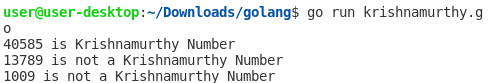## Posts

Showing posts with the label Krishnamurthy number

### Go Language - Program to Check Krishnamurthy NumberHello everyone, today we will create a Go program to check if the given number is an Krishnamurthy number or not. Krishnamurthy number is also referred to as a Strong number. A number is said to be Krishnamurthy if the factorial sum of all its digits is equal to that number. For example, Number = 40585   = 4! + 0! + 5! + 8! + 5!   = 1 * 2 * 3 * 4 + 1 + 1 * 2 * 3 * 4 * 5 +  1 * 2 * 3 * 4 * 5 * 6 * 7 * 8 +  1 * 2 * 3 * 4 * 5   = 24 + 1 + 120 +  40320 + 120   =  40585 Example 1: Go program that checks if the given number is a Krishnamurthy number or not. /*Go program to check whether the given * number is a Krishnamurthy number or not. */ package main import "fmt" // Main function func main() { //sample 1 number1 := 40585 KrishnamurthyNumber(number1) //sample 2 number2 := 13789 KrishnamurthyNumber(number2) //sample 3 number3 := 1009 KrishnamurthyNumber(number3) } func KrishnamurthyNumber(number int ) { //initialize sum to 0 sum